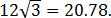Equable Bicone

EQUABLE BICONE

Balmoral Software

Solutions: 1 (rational height)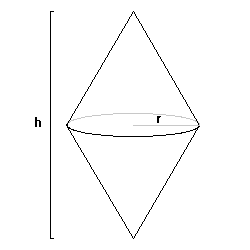A bicone is a solid of revolution created by rotating a rhombus around one of its diagonals. There is exactly one equable bicone with a rational height and rational square of radius.

Proof. For an equable bicone with radius r and overall height h, we have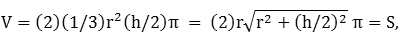which reduces to
 (r2 - 9)h2 = 36r2, r > 3 
for an equable solution. By convention, W = V/π = r2h/3 is a positive integer. Then r2 = 3W/h > 9, so h < W/3 and  can be written
(3W/h - 9)h2 = 36(3W/h)

3h3 - Wh2 + 36W = 0, 0 < h < W/3

The equable solutions for h are the zeroes of the cubic
fW(h) = 3h3 - Wh2 + 36W
Since
d/dh fW(h) = 9h2 - 2Wh
and
d2/dh2 fW(h) = 18h - 2W,
fW has critical points at h = 0 and h = 2W/9 > 0. The first is a relative maximum and the second a relative minimum, and fW(0) = 36W > 0. Therefore, there are two positive real zeroes when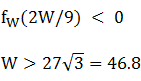In conclusion, there are infinitely-many equable bicones with real dimensions, and volumes that are integers of at least 47, times π.

### Rational height

Now assume h is rational. Solving  for r2, we have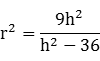and any equable solution requires that h > 6. It follows that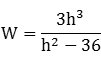We will show that h must be an integer. Let the height h = N/D for positive integers N and D. Since h > 6,
 D < N/6, 
and we can write  as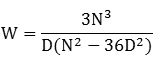Each factor in the denominator divides the numerator. Since D < N by , then if D divides N3, it also divides N and h is an integer. Otherwise, D divides 3. If D = 1, then h is again an integer, so assume D = 3. Then  becomes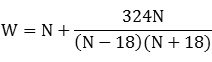Since h = N/3 > 6, we have N > 18 and the fraction on the right is a positive integer, so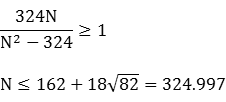Each factor of the denominator in  divides the numerator (this is a necessary but not sufficient condition for W to be an integer). By long division,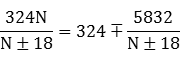so both N - 18 and N + 18 are factors of 5832, subject to 19 ≤ N ≤ 324:
N ∈ {19,20,21,22,24,26,27,30,36,42,45,54,72,90,99,126,180,234,261} ⋂ {36,54,63,90,144,198,225,306} = {36,54,90}

h = N/3 ∈ {12,18,30}

Evaluating in , the only solution for which W is an integer is h = 12. From , the corresponding value for r2 is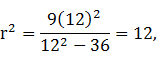so r is irrational. In this case, the volume and surface area are 48π. That bicone is a solid of revolution of a non-equable isosceles triangle with base 12 and height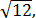having perimeter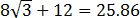and area